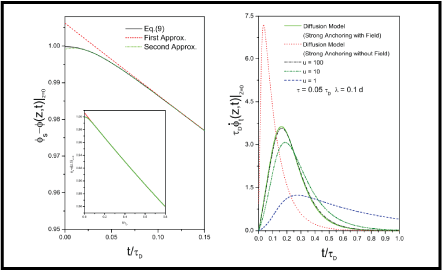# Inﬂuence of the anchoring energy on the relaxation of the nematic deformation### Abstract

We analyze the inﬂuence of the anchoring energy strength on the relaxation of the nematic deformation, when the distorting ﬁeld is removed, at t = 0. The analysis is performed by assuming that the nematic sample is in the shape of a slab, the anchoring energy can be approximated by the form proposed by Rapini and Papoular, and the surface dissipation, responsible for the surface viscosity, is negligible with respect to the bulk one. The switching time of the distorting ﬁeld is supposed ﬁnite, to avoid the non-physical discontinuity of the time derivative of the tilt angle at t = 0. We show that the relaxation time of the nematic deformation is a multi-relaxation phenomenon. For large t, the relaxation phenomenon is simple, and the relaxation time is proportional to the diﬀusion time τ_D = ηd^2/k, where d is the thickness of the sample, η the viscosity and k the elastic constant of the nematic liquid crystal. For t≪τ_D, the time dependence of the tilt angle is well approximated by few exponential terms. The relation between the eﬀective relaxation time and the anchoring energy strength is deduced. A critical discussion on the standard analysis based on the diﬀusion equation is also reported.

Type